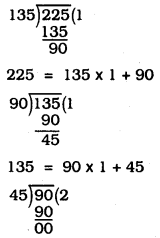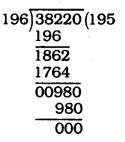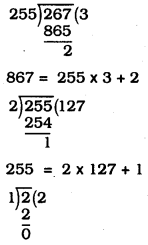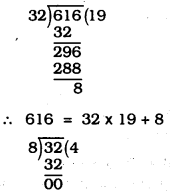KSEEB SSLC Class 10 Maths Solutions Chapter 8 Real Numbers Ex 8.1

In this chapter, we provide KSEEB SSLC Class 10 Maths Solutions Solutions Chapter 8 Real Numbers Ex 8.1 for English medium students, Which will very helpful for every student in their exams. Students can download the latest KSEEB SSLC Class 10 Maths Solutions Solutions Chapter 8 Real Numbers Ex 8.1 pdf, free KSEEB SSLC Class 10 Maths Solutions Solutions Chapter 8 Real Numbers Ex 8.1 pdf download. Now you will get step by step solution to each question.

Karnataka State Syllabus Class 10 Maths SolutionsSolutions Chapter 8 Real Numbers Ex 8.1

Question 1.
Use Euclid’s division algorithm to find the HCF of:
(i) 135 and 225
(ii) 196 and 38220
(iii) 867 and 255
Solution:
(i) 135 and 225∴ 90 = 45 × 2 + 0
∴ HCF of 135 and 225 is 45.

(ii) 196 and 38220∴ 38220 = 196 × 195 + 0
∴ HCF of 196 and 38220 is 196.

(iii) 867 and 25522 = 1 × 2 + 0
∴ HCF of 867 and 2552 is 1.

Question 2.
Show that any positive odd integer is of the form 6q + 1, or 6q + 3, or 6q + 5, where q is some integer.
Solution:
Let us consider a positive odd integer as ‘a’.
On dividing ‘a’ by 6, let q be the quotient and ‘r’ be the remainder.
∴ Using Euclid’s lemma, we get a = 6q + r
where 0 ≤ r < 6 i.e., r = 0, 1, 2, 3, 4 or 5 i.e.,
a = 6q + 0 = 6q or a = 6q + 1
or a = 6q + 2 or a = 6q + 3
or a = 6q + 4 or a = 6q + 5
But, a = 6q, a = 6q + 2, a = 6q + 4 are even values of ‘a’.
[∵ 6q = 2(3q) = 2m1 6q + 2 = 2(3q + 1) = 2m2,
6q + 4 = 2(3 q + 2) = 2m3]
But ‘a’ being an odd integer, we have :
a = 6q + 1, or a = 6q + 3, or a = 6q + 5

Question 3.
An army contingent of 616 members is to march behind an army band of 32 members in a parade. The two groups are to march in the same number of columns. What is the maximum number of columns in which they can march ?
Solution:
HCF of 32 and 616∴ 32 = 8 × 4 + 0
∴ HCF of 32 and 616 is 8.
∴ Both groups can march in 8 columns.

Question 4.
Use Euclid’s division lemma to show that the square of any positive integer is either of the form 3m or 3m + 1 for some integer m.
[Hint: Let x be any positive integer then it is of the form 3g, 3q + 1 or 3g + 2. Now square each of these and show that they can be rewritten in the form 3m or 3m +1.]
Solution:
Let us consider an arbitrary positive integer as ‘x’ such that it is of the form
3q, (3q + 1) or (3q + 2)
For x = 3q, we have x2 = (3q)2
⇒ x2 = 9q2 = 3(3q2) = 3m ………. (1)
Putting 3q2 = m, where m is an integer.
For x = 3q + 1,
x2 = (3q + 1)2 = 9q2 + 6q + 1
= 3(3q2 + 2q) + 1 = 3m + 1 ………… (2)
Putting 3q2 + 2q = m, where m is an integer.
For x = 3q + 2,
x2 = (3q + 2)2
= 9q2 + 12q + 4 = (9q2 + 12q + 3) + 1
= 3(3q2 + 4q + 1) + 1 = 3m + 1 ……….. (3)
Putting 3q2 + 4q +1 = m, where m is an integer.
From (1), (2) and (3),
x2 = 3m or 3m + 1
Thus, the square of any positive integer is either of the form 3m or 3m + 1 for some integer m.

Question 5.
Use Euclid’s division lemma to show that the cube of any positive integer is of the form 9m, 9m + 1 or 9m + 8.
Solution:
Let a and b be two positive integers and a > b
a = (b × q) + r where q and r are positive integers and 0 ≤ r < b.
Let b = 3 (if 9 is multiplied by 3 a perfect cube number is obtained)
a = 3q + r where 0 ≤ r < 3
(i) if r = 0, a = 3q
(ii) if r = 1, a = 3q + 1
(iii) if r = 2 a = 3q + 2
Consider cubes of these,
case i) a = 3q
a3 = (3q)3 = 27q3 = 9(3q3)
a3 = 9m
where m= 3q3 & m is an integer

case ii) a = 3q + 1
a3 = (3q + 1)3
(a + b)3 = a3 + b3 + 3a2b + 3ab2
a3 = 27q3 + 1 + 27q3 + 9q
a3 = 27 q3 + 27 q2 + 9q + 1
a3 = 9 (3q3 + 3q2 + q) + 1
a3 = 9m + 1
where m = 3q3 + 3q2 + q & m is an integer

case iii) a = 3q + 2
a3 = (3q + 2)3 = 27q3 + 8 + 54q2 + 36q
a3 = 27q3 + 54q2 + 36q + 8
= 9 (3q3 + 6q2 + 4q) + 8
a3 = 9m + 8
where m = 3q3 + 6q2 + 4q and m is an integer.
∴ Cube of any positive integer is either of the form 9m, 9m + 1 (or) 9m + 8 for some integer m.

All Chapter KSEEB Solutions For Class 10 Maths

—————————————————————————–

All Subject KSEEB Solutions For Class 10

*************************************************

I think you got complete solutions for this chapter. If You have any queries regarding this chapter, please comment on the below section our subject teacher will answer you. We tried our best to give complete solutions so you got good marks in your exam.

If these solutions have helped you, you can also share kseebsolutionsfor.com to your friends.

Best of Luck!!